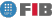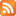Saltar al contingut Menu

# Discrete Mathematics (MATD)

Credits Dept. Type Requirements
9.0 (7.2 ECTS) MAT
• Compulsory for DIE
• Elective for DCSFW
• Elective for DCSYS
AL - Prerequisite for DIE , DCSYS , DCSFW

## Instructors

 Person in charge: (-) Others: (-)

## General goals

The overall aim of this subject is to introduce students to the concepts and techniques involved in discrete mathematics and algebra that, due to their ubiquity in the world of new technologies, form part of the basic training of any engineer in information technology.

## Specific goals

### Knowledges

1. Learn the basic principles of enumeration: principles of identify, product, Pigeon-hole Principle, inclusion-exclusion. Learn the algebra of generating functions.

Learn how to express recurrences in terms of generating functions.
2. Learn graph and digraph structure as a mathematical model and the various forms that graphs may take.

Learn tree characterisations and obtain tree generators at minimum cost.
3. Learn the characterisations of Eulerian graphs and, where applicable, how to obtain a Eulerian path where one exists. Learn the problem posed by Hamiltonian circles, and associated difficulties.
4. Learn the concepts of flux, connectivity, and pairing, and the following basic theorems: maximum flow/minimum cut theorem, Menger theorems, Hall theorems.
5. Learn the structure of a field in terms of Z_p (integer module, prime p) and the ring of polynomials with coefficients Z_p. Learn what an irreducible polynomial is and learn the single factorisation theorem. Learn how to construct finite field and ascertain their existence and unicity.

### Abilities

1. Ability to apply the basic principles of enumeration. Ability to work with generating functions. Ability to frame enumeration problems in terms of recurrences. Ability to solve recurrences by employing generating functions.
2. Ability to model problems in terms of graphs and digrafs. Learn how to use the relationships linking the parameters of a planar graph in order to deduce the values for some parameters from the values of others.

Ability to calculate the number of tree generators in a connection graph. Ability to apply research algorithms in depth and breadth to obtain tree generators. Ability to apply Prim algorithms and Kruskal algorithms to obtain tree generators at minimum cost.
3. Ability to apply algorithms to obtain a Eulerian cycle and a Eulerian circuit. Ability to apply sufficient Hamiltonian conditions to simple examples. Ability to operate with cycles.
4. Ability to apply the Ford and Fulkerson to obtain maximum flux.

Ability to apply Menger theorems and Hall theorems in simple situations.
5. Learn how to operate Z_p with p prime, and in particular, how to calculate inverse matrices.

Ability to operate with polynomials with Z_p coefficients. Ability to construct finite bodies.

Ability to operate with finite bodies, using the polynomial form of its elements, and using discrete logarithms. Ability to operate with polynomials in a finite body.

### Competences

1. Critical, logical-mathematical reasoning.
2. Ability to apply techniques for constructing logical-mathematical demonstrations.
3. Ability to transform informal problems into formal ones and vice versa.
4. Ability to apply mathematical knowledge and logic in solving problems.
5. Ability to think in abstract terms. Ability to tackle new problems by consciously using strategies that have proved useful in solving previous problems.

## Contents

Estimated time (hours):

 T P L Alt Ext. L Stu A. time Theory Problems Laboratory Other activities External Laboratory Study Additional time

1. Principles of enumeration
T      P      L      Alt    Ext. L Stu    A. time Total
8,0 8,0 0 0 0 21,0 0 37,0
Principles of equality, addition and product. Binomial numbers. Multisets. Multinomial numbers. Catalan names. Integer partitions. Inclusion-exclusion principle. The Pigeonhole Principle (Dirichlet"s Box Principle).

2. Generating functions
T      P      L      Alt    Ext. L Stu    A. time Total
7,0 7,0 0 0 0 19,0 0 33,0
Partial fractions. Successions and generating functions. Linear recurrences. Catalan names. Partitions. Exponential generating function. Disarrangements. Stirling numbers and Bell numbers.

3. Graphs
T      P      L      Alt    Ext. L Stu    A. time Total
8,0 8,0 0 0 0 21,0 0 37,0
Definitions. Isomorphism. Eulerian graphs and matching lemma. Circuits, paths, distance, connections and connectedness. Operations with graphs. Eulerian graphs. Hamiltonian graphs. Matricial representation of a graph.

4. Trees
T      P      L      Alt    Ext. L Stu    A. time Total
6,0 7,0 0 0 0 18,0 0 31,0
Definition and characterisation of trees. Tree generators. Obtention through broad and in-depth searches. Number of tree generators in a graph. Minimal tree generators. Kruskal"s algorithm and Prim"s algorithm.

5. Polynomials and finite bodies.
T      P      L      Alt    Ext. L Stu    A. time Total
7,0 8,0 0 0 0 20,0 0 35,0
Z_p rings of residue class modules of an integer prime. Polynomials rings with Z_p coefficients.

Greatest common divisor and Bezout"s identity. Irreducible polynomials and unique factorisation.

Roots. Polynomial module quotients. Construction of finite bodies. Discrete logarithms.

Polynomials over finite fields.

 Total per kind T P L Alt Ext. L Stu A. time Total 36,0 38,0 0 0 0 99,0 0 173,0 Avaluation additional hours 7,0 Total work hours for student 180,0

## Docent Methodolgy

Theory classes will take the form of lectures, which may include the use of an overhead slide projector or computer demonstrations.

The classes of problems will be of a participatory nature, with students explaining previously set problems and discussing other previously set problems in class.

## Evaluation Methodgy

The evaluation is continuous, based on the following three exams:

P1: covering the initial part of the course,
P2: covering the middle part of the course,
P3: covering the final part of the course.

The final grade is N= 0.2*P1+0.4*P2+0.4*P3 (where P1,P2,P3
are grades over 10).

The students who do not want to follow the continuous evaluation or wish
to renounce to it, must take an exam covering the whole course, and
their final grade will be the one obtained in this exam. The student
must inform the coordinator about the renounce by the end of the
classes, following the procedure that will be posted on the Raco.

## Basic Bibliography

• Francesc Comellas ... [et al.] Matemática discreta, Edicions UPC, 2001.
• Norman L. Biggs Matemática discreta, Vicens-Vives, 1994.
• Ralph P. Grimaldi Matemáticas discreta y combinatoria : una introducción con aplicaciones, Addison-Wesley Iberoamericana, 1997.

## Complementary Bibliography

• Josep M. Brunat Combinatòria i teoria de grafs, Edicions UPC, 1997.
• Joan Trias Pairó Matemàtica discreta : problemes resolts, Edicions UPC, 2001.
• Jiri Matousek and Jaroslav Nesetril Invitation to discrete mathematics, Clarendon Press : Oxford University Press, 1998.
• Kenneth H. Rosen Matemática discreta y sus aplicaciones, McGraw-Hill, 2004.

(no available informacion)

## Previous capacities

Understand operations with and relationships in sets: union, intersection, difference, Cartesian product, inclusion.

Understand the various applications of sets.

Ability to count combinations and permutations (with and without repetition).

Understand the basic properties of binomial numbers and how to calculate them.

Know how to calculate the GCD (Greatest Common Denominator) of integers and Bezout identity coefficients using Euclides" algorithm.

Know how to calculate the products of matrices and how to calculate determinants.

AL is a prerequisite for taking this course.© Barcelona school of informatics - Contact -RSS
This website uses cookies to offer you the best experience and service. If you continue browsing, it is understood that you accept our cookies policy.
Classic version Mobile version